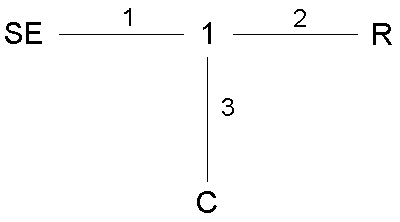Bondgraph Analysis Program Synthesis

BAPS is a program system for presenting and analysing bondgraphs.

BAPS analyses linear and nonlinear bondgraphs and assigns causalities.

BAPS converts a BAPS-program into a simulation model, e.g. for SIMUL_R.

BAPSDRAW allows for the graphical creation of BAPS-programs.

The BAPS-language

describes the bondgraph in bond-notation in a textual way.

• Element-constants can be formulated as constants or nonlinearities,
• the constituting nonlinear equations of elements can be specified directly,
• using logical switches time variant value and topological changes (in the bond connections) can be described,
• table functions can be used.

Modern modelling methods

BAPS support the bondgraph-modelling by

• possibilities for partitioning in connected and disconnected partial graphs
• rapid prototyping in partial models,
• meta commands, like macros and text loops,
• language elements for using parallel computing simulation systems
• inclusion of partial differential equations (simulated using SIMUL_R™).

The BAPS-compiler

• performs causality checks and -assignments,
• checks linear and nonlinear systems for algebraic loops and
• creates optimized simulation models.

Simple example: RC-swinger/spring-damping system

Bondgraph:BAPS-model:

RC_System:

SE 1; 1 1 2 3; R 2; C 3;

{

CONSTANT tend=0.05, frequ=50, f=frequ*2*3.14, ampl=220;

EXTERN sin(1);

"termination condition"

TERMINATE t>=tend;

"element constants"

SE 1:     SE1=ampl*sin(t*f);

R 2:      R2=100;

C 3:     C3=0.0001;     q03=0; "initial charge"

}

BAPS is available on Windows- and Unix-plattforms for the simulation languages SIMUL_R™, ACSL™, Matlab™, Simulink™, Hybsys.

Use the info-form to request further information.

 Home Copyright Simutech 2002 Mail to webmaster